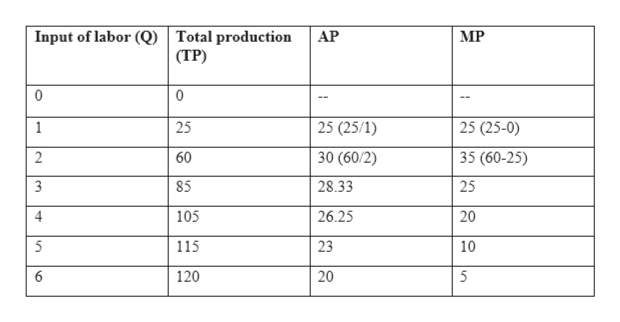# The short-run production function for a manufacturer of flash memory drives is shown in the table below. Based on this information, answer the following questions. Input of Labor (workers per week)Total Output of Flash Memory Drives00125260385410551156120a.   Calculate the average product at each quantity of labor.b.   Calculate the marginal product of labor at each quantity of labor.c.   At what point does marginal product begin to diminish?

Question
28 views

The short-run production function for a manufacturer of flash memory drives is shown in the table below. Based on this information, answer the following questions.

 Input of Labor (workers per week) Total Output of Flash Memory Drives 0 0 1 25 2 60 3 85 4 105 5 115 6 120

a.   Calculate the average product at each quantity of labor.

b.   Calculate the marginal product of labor at each quantity of labor.

c.   At what point does marginal product begin to diminish?

check_circle

Step 1

a) Average product is the output produced per unit of labor.

Formula for calculating average product:

Step 2

Step3:

b) Marginal product can be calculated as the change in total output on addition of one more unit of labor input.

The formula for calculating marginal product:

Step 3

The following table shows average product and ma...help_outlineImage TranscriptioncloseInput of labor (Q) Total production (ТР) АР МP 25 (25/1) 25 (25-0) 25 1 30 (60/2) 2 60 35 (60-25) 85 28.33 105 26.25 20 5 23 10 115 120 5 25 20 4 fullscreen

### Want to see the full answer?

See Solution

#### Want to see this answer and more?

Solutions are written by subject experts who are available 24/7. Questions are typically answered within 1 hour.*

See Solution
*Response times may vary by subject and question.
Tagged in